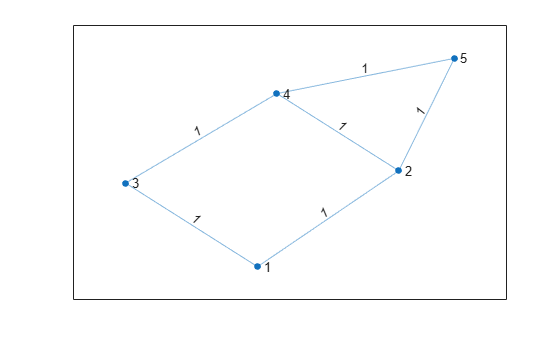# Calculate Number of Lines of Code by Using Report Information Object

This example shows how to calculate the number of lines in the source code and the generated code by using the report information object. For more information on the report information object, see Access Code Generation Report Information Programmatically.

### Setup

```path = fullfile(matlabroot, 'examples', 'coder', 'main'); addpath(path);```

### The MATLAB Code

In this example, you generate code for the MATLAB function `dijkstra`. This function calculates the lengths of the shortest paths from a node to every other node in a graph by using Dijkstra's algorithm.

`type dijkstra`
```% DIJKSTRA Find length of shortest path between nodes in a graph % % D = dijkstra(A, p) % Takes a graph represented by its adjacency matrix 'A' along with a node % 'p' as input and returns a vector 'D' containing the length of the % shortest path from 'p' to all other nodes in the graph. % Copyright 2018 The MathWorks, Inc. function D = dijkstra(A, p) %#codegen narginchk(2,2); [m, n] = size(A); % Assertions to make sure inputs are valid assert(m == n, "Input adjacency matrix for graph must be a square matrix"); assert(rem(p, 1) == 0 && p <= m && p > 0, "Input src must be a node in the graph"); % Initialization max = realmax; D = repmat(max, 1, m); D(p) = 0; visited = false(1, m); for i = 1:m % Select next node to visit min = max; u = -1; for v = 1:n if ~visited(v) && D(v) <= min min = D(v); u = v; end end % Mark selected node as visited visited(u) = true; %{ Update distances of nodes adjacent to selected node that are yet to be visited %} for v = 1:n if(~visited(v) && A(u, v) ~= 0 && D(u) ~= max) distVal = D(u) + A(u, v); if distVal < D(v) D(v) = distVal; end end end end end ```

Specify the adjacency matrix `A` for a graph and a node `p` where the traversal of the graph begins. Plot the graph. Call `dijkstra` to compute the shortest distance from `p` to every node in the graph and display these distances.

```% Sample adjacency Matrix for graph with 5 nodes A = [ 0 1 1 0 0; 1 0 0 1 1; 1 0 0 1 0; 0 1 1 0 1; 0 1 0 1 0 ]; % Plot the graph to see how it looks like G = graph(A, 'omitselfloops'); plot(G, 'EdgeLabel', G.Edges.Weight)``````% Source node from where graph traversal begins p = randi(size(A, 1)); % Calculate shortest distance from 'p' to every other node in graph G D = dijkstra(A, p); for i=1:numel(D) fprintf("Length of shortest path from %d to %d is %d. \n", p, i, D(i)); end```
```Length of shortest path from 5 to 1 is 2. Length of shortest path from 5 to 2 is 1. Length of shortest path from 5 to 3 is 2. Length of shortest path from 5 to 4 is 1. Length of shortest path from 5 to 5 is 0. ```

### Export Information about Code Generation

The report information object provides programmatic access to information about code generation. The properties of this object provide information about code generation settings, input files, generated files, and code generation error, warning, and information messages.

To export the report information object to a variable in your base MATLAB workspace, include the `-reportinfo` option with the name of the variable while running the codegen command. In this example, you export the code generation report information to the variable `info`.

`codegen -c dijkstra -args {A, p} -reportinfo info`

### Calculate Number of Lines of Code

The `loc` function takes a report information object as input and returns two outputs that contain the number of lines in the source code and the generated code, respectively. This function excludes blank lines and the lines containing comments while computing the number of lines of code.

`type loc`
```% LOC Calculate total lines of source and generated code in a codegen run % % [i, o] = loc(r) % Takes a report information object 'r' as input, and produces two % outputs - 'i' and 'o' containing the total lines of code in the source % MATLAB files and generated files respectively. % Copyright 2018 The MathWorks, Inc. function [i, o] = loc(r) narginchk(1,1); % Assert that input is a report information object. assert(isa(r, 'coder.ReportInfo'), 'Input must be of type coder.ReportInfo'); % Fetch source and generated files from the report information object. sourceFiles = r.InputFiles; generatedFiles = r.GeneratedFiles; % Count lines of code in source and generated files. Blank lines, and % comments are not counted. i = countLines(sourceFiles, true); o = countLines(generatedFiles, false); end function count = countLines(files, isSource) count = 0; for i=1:numel(files) f = files(i); if isprop(f, 'Text') lines = splitlines(f.Text); for j=1:numel(lines) line = strtrim(lines{j}); if ~isempty(line) && ~isComment(line, isSource) count = count + 1; end end clear isComment; % clear persistent variables end end end function result = isComment(line, isSource) persistent inBlockComment; if isempty(inBlockComment) inBlockComment = false; end if isSource result = (startsWith(line, "%") || inBlockComment); if line == "%{" || line == "%}" inBlockComment = (line ~= "%}"); end else result = (startsWith(line, "/") || inBlockComment); if startsWith(line, "/*") || endsWith(line, "*/") inBlockComment = ~endsWith(line, "*/"); end end end ```

Call `loc` with the report information object `info` as input. Display the number of lines of code in the source files and the generated files.

```info = evalin('base', 'info'); [nLocIn, nLocOut] = loc(info); fprintf('Lines of code in source MATLAB file(s): %d', nLocIn);```
```Lines of code in source MATLAB file(s): 29 ```
`fprintf('Lines of code in generated file(s): %d', nLocOut);`
```Lines of code in generated file(s): 568 ```

### Cleanup

Remove example files from path.

`rmpath(path);`